GATE  >  Computer Science And Information Technology - CS 2013 GATE Paper (Practice Test)

# Computer Science And Information Technology - CS 2013 GATE Paper (Practice Test) - GATE

Test Description

## 65 Questions MCQ Test GATE Past Year Papers for Practice (All Branches) - Computer Science And Information Technology - CS 2013 GATE Paper (Practice Test)

Computer Science And Information Technology - CS 2013 GATE Paper (Practice Test) for GATE 2023 is part of GATE Past Year Papers for Practice (All Branches) preparation. The Computer Science And Information Technology - CS 2013 GATE Paper (Practice Test) questions and answers have been prepared according to the GATE exam syllabus.The Computer Science And Information Technology - CS 2013 GATE Paper (Practice Test) MCQs are made for GATE 2023 Exam. Find important definitions, questions, notes, meanings, examples, exercises, MCQs and online tests for Computer Science And Information Technology - CS 2013 GATE Paper (Practice Test) below.
Solutions of Computer Science And Information Technology - CS 2013 GATE Paper (Practice Test) questions in English are available as part of our GATE Past Year Papers for Practice (All Branches) for GATE & Computer Science And Information Technology - CS 2013 GATE Paper (Practice Test) solutions in Hindi for GATE Past Year Papers for Practice (All Branches) course. Download more important topics, notes, lectures and mock test series for GATE Exam by signing up for free. Attempt Computer Science And Information Technology - CS 2013 GATE Paper (Practice Test) | 65 questions in 180 minutes | Mock test for GATE preparation | Free important questions MCQ to study GATE Past Year Papers for Practice (All Branches) for GATE Exam | Download free PDF with solutions
 1 Crore+ students have signed up on EduRev. Have you?
Computer Science And Information Technology - CS 2013 GATE Paper (Practice Test) - Question 1

### Q. No. 1 – 5 Carry One Mark Each Q. Complete the sentence: Universalism is to particularism as diffuseness is to ________

Detailed Solution for Computer Science And Information Technology - CS 2013 GATE Paper (Practice Test) - Question 1

The relation is that of antonyms

Computer Science And Information Technology - CS 2013 GATE Paper (Practice Test) - Question 2

### Were you a bird, you ___________ in the sky.

Computer Science And Information Technology - CS 2013 GATE Paper (Practice Test) - Question 3

### Which one of the following options is the closest in meaning to the word given below? Nadir

Detailed Solution for Computer Science And Information Technology - CS 2013 GATE Paper (Practice Test) - Question 3

Nadir in the lowest point on a curve

Computer Science And Information Technology - CS 2013 GATE Paper (Practice Test) - Question 4

Choose the grammatically INCORRECT sentence:

Computer Science And Information Technology - CS 2013 GATE Paper (Practice Test) - Question 5

What will be the maximum sum of 44, 42, 40, ... ?

Detailed Solution for Computer Science And Information Technology - CS 2013 GATE Paper (Practice Test) - Question 5

The maximum sum is the sum of 44, 42,- - - - -2.
The sum of ‘n’ terms of an AP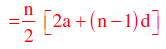In this case, n = 22, a = 2 and d = 2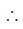Sum =11[4 + 21×2] =11×46 = 506

Computer Science And Information Technology - CS 2013 GATE Paper (Practice Test) - Question 6

Q. No. 6 – 10 Carry Two Marks Each

Q.

Out of all the 2-digit integers between 1 and 100, a 2-digit number has to be selected at
random. What is the probability that the selected number is not divisible by 7?

Detailed Solution for Computer Science And Information Technology - CS 2013 GATE Paper (Practice Test) - Question 6

The number of 2 digit multiples of 7 = 13Probability of choosing a number
Not divisible by 7 =(90-13)/90 =77/90

Computer Science And Information Technology - CS 2013 GATE Paper (Practice Test) - Question 7

A tourist covers half of his journey by train at 60 km/h, half of the remainder by bus at 30
km/h and the rest by cycle at 10 km/h. The average of the tourist in km/h during his entire
journey is

Detailed Solution for Computer Science And Information Technology - CS 2013 GATE Paper (Practice Test) - Question 7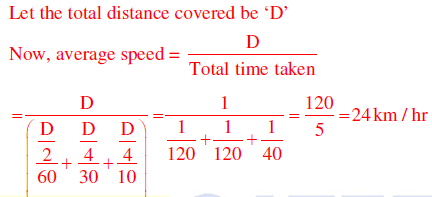Computer Science And Information Technology - CS 2013 GATE Paper (Practice Test) - Question 8

Find the sum of the expression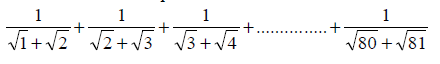Detailed Solution for Computer Science And Information Technology - CS 2013 GATE Paper (Practice Test) - Question 8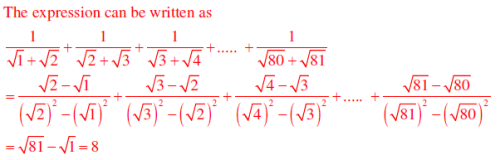Computer Science And Information Technology - CS 2013 GATE Paper (Practice Test) - Question 9

The current erection cost of a structure is Rs. 13,200. If the labour wages per day increase by
1/5 of the current wages and the working hours decrease by 1/24 of the current period, then
the new cost of erection in Rs. is

Detailed Solution for Computer Science And Information Technology - CS 2013 GATE Paper (Practice Test) - Question 9

Let ‘W’ be the labour wages, and ‘T’ be the working hours.
Now, total cost is a function of W× T
Increase in wages = 20%Revised wages = 1.2 W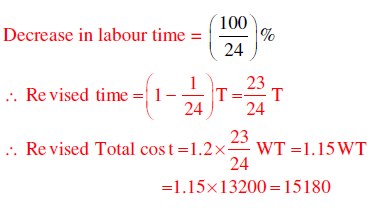Computer Science And Information Technology - CS 2013 GATE Paper (Practice Test) - Question 10

After several defeats in wars, Robert Bruce went in exile and wanted to commit suicide. Just
before committing suicide, he came across a spider attempting tirelessly to have its net. Time
and again, the spider failed but that did not deter it to refrain from making attempts. Such
attempts by the spider made Bruce curious. Thus, Bruce started observing the nearimpossible
goal of the spider to have the net. Ultimately, the spider succeeded in having its
net despite several failures. Such act of the spider encouraged Bruce not to commit suicide.
And then, Bruce went back again and won many a battle, and the rest is history.

Which one of the following assertions is best supported by the above information?

Computer Science And Information Technology - CS 2013 GATE Paper (Practice Test) - Question 11

Q. No. 11 – 35 Carry One Mark Each

Q.

Consider an undirected random graph of eight vertices. The probability that there is an edge
between a pair of vertices is ½. What is the expected number of unordered cycles of length
three?

Detailed Solution for Computer Science And Information Technology - CS 2013 GATE Paper (Practice Test) - Question 11

P(edge) =1/2
Number of ways we can choose the vertices out of 8 is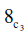(Three edges in each cycle)
Expected number of unordered cycles of length 3 =x (1/2)3 =7

Computer Science And Information Technology - CS 2013 GATE Paper (Practice Test) - Question 12

Which of the following statements is/are TRUE for undirected graphs?
P: Number of odd degree vertices is even.
Q: Sum of degrees of all vertices is even.

Detailed Solution for Computer Science And Information Technology - CS 2013 GATE Paper (Practice Test) - Question 12

Q: Sum of degrees of all vertices = 2×(number of edges)

Computer Science And Information Technology - CS 2013 GATE Paper (Practice Test) - Question 13

Function f is known at the following points: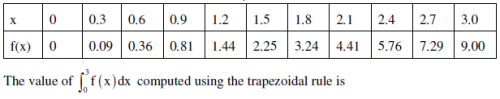Detailed Solution for Computer Science And Information Technology - CS 2013 GATE Paper (Practice Test) - Question 13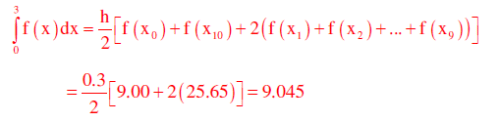Computer Science And Information Technology - CS 2013 GATE Paper (Practice Test) - Question 14

Which one of the following functions is continuous at x = 3 ?

Detailed Solution for Computer Science And Information Technology - CS 2013 GATE Paper (Practice Test) - Question 14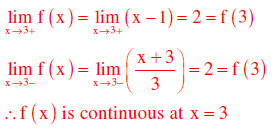Computer Science And Information Technology - CS 2013 GATE Paper (Practice Test) - Question 15

Which one of the following expressions does NOT represent exclusive NOR of x and y?

Detailed Solution for Computer Science And Information Technology - CS 2013 GATE Paper (Practice Test) - Question 15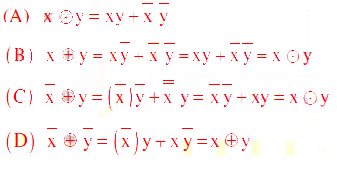Computer Science And Information Technology - CS 2013 GATE Paper (Practice Test) - Question 16

In a k-way set associative cache, the cache is divided into v sets, each of which consists of k
lines. The lines of a set are placed in sequence one after another. The lines in set s are
sequenced before the lines in set (s+1). The main memory blocks are numbered 0 onwards.
The main memory block numbered j must be mapped to any one of the cache lines from

Detailed Solution for Computer Science And Information Technology - CS 2013 GATE Paper (Practice Test) - Question 16

Position of main memory block in the cache (set) = (main memory block number) MOD
(number of sets in the cache).
As the lines in the set are placed in sequence, we can have the lines from 0 to (K – 1) in each
set.
Number of sets = v, main memory block number = j
First line of cache = (j mod v)*k; last line of cache = (j mod v)*k + (k – 1)

Computer Science And Information Technology - CS 2013 GATE Paper (Practice Test) - Question 17

What is the time complexity of Bellman-Ford single-source shortest path algorithm on a
complete graph of n vertices?

Detailed Solution for Computer Science And Information Technology - CS 2013 GATE Paper (Practice Test) - Question 17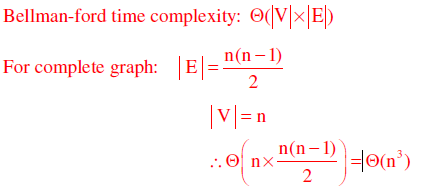Computer Science And Information Technology - CS 2013 GATE Paper (Practice Test) - Question 18

Which of the following statements are TRUE?
(1) The problem of determining whether there exists a cycle in an undirected graph is in P.
(2) The problem of determining whether there exists a cycle in an undirected graph is in NP.
(3) If a problem A is NP-Complete, there exists a non-deterministic polynomial time
algorithm to solve A.

Detailed Solution for Computer Science And Information Technology - CS 2013 GATE Paper (Practice Test) - Question 18

1. Cycle detection using DFS:  O(V+ E) = O(V2) and it is polynomial problem
2. Every P-problem is NP(since P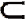NP)
3. NP − complete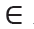NP
Hence, NP-complete can be solved in non-deterministic polynomial time

Computer Science And Information Technology - CS 2013 GATE Paper (Practice Test) - Question 19

Which of the following statements is/are FALSE?
(1) For every non-deterministic Turing machine, there exists an equivalent deterministic
Turing machine.
(2) Turing recognizable languages are closed under union and complementation.
(3) Turing decidable languages are closed under intersection and complementation
(4) Turing recognizable languages are closed under union and intersection.

Detailed Solution for Computer Science And Information Technology - CS 2013 GATE Paper (Practice Test) - Question 19

(1) NTM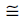DTM
(2) RELs are closed under union & but not complementation
(3) Turing decidable languages are recursive and recursive languages are closed under
intersection and complementation
(4) RELs are closed under union & intersection but not under complementation

Computer Science And Information Technology - CS 2013 GATE Paper (Practice Test) - Question 20

Three concurrent processes X, Y, and Z execute three different code segments that access and
update certain shared variables. Process X executes the P operation (i.e., wait) on semaphores
a, b and c; process Y executes the P operation on semaphores b, c and d; process Z executes
the P operation on semaphores c, d, and a before entering the respective code segments. After
completing the execution of its code segment, each process invokes the V operation (i.e.,
signal) on its three semaphores. All semaphores are binary semaphores initialized to one.
Which one of the following represents a deadlock-free order of invoking the P operations by
the processes?

Detailed Solution for Computer Science And Information Technology - CS 2013 GATE Paper (Practice Test) - Question 20

Suppose X performs P(b) and preempts, Y gets chance, but cannot do its first wait i.e., P(b),
so waits for X, now Z gets the chance and performs P(a) and preempts, next X gets chance.
X cannot continue as wait on ‘a’ is done by Z already, so X waits for Z. At this time Z can
continue its operations as down on c and d. Once Z finishes, X can do its operations and so Y.
In any of execution order of X, Y, Z one process can continue and finish, such that waiting is
not circular. In options (A),(C) and (D) we can easily find circular wait, thus deadlock

Computer Science And Information Technology - CS 2013 GATE Paper (Practice Test) - Question 21

An index is clustered, if

Detailed Solution for Computer Science And Information Technology - CS 2013 GATE Paper (Practice Test) - Question 21

Clustered index is built on ordering non key field and hence if the index is clustered then the
data records of the file are organized in the same order as the data entries of the index.

Computer Science And Information Technology - CS 2013 GATE Paper (Practice Test) - Question 22

Assume that source S and destination D are connected through two intermediate routers
labeled R. Determine how many times each packet has to visit the network layer and the data
link layer during a transmission from S to D.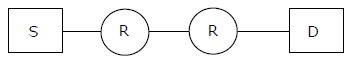Detailed Solution for Computer Science And Information Technology - CS 2013 GATE Paper (Practice Test) - Question 22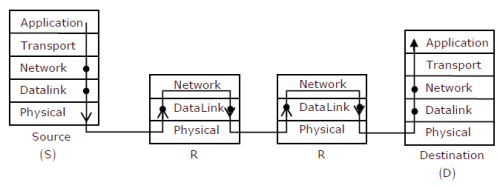From above given diagram, its early visible that packet will visit network layer 4 times, once
at each node [S, R, R, D] and packet will visit Data Link layer 6 times. One time at S and one
time at D, then two times for each intermediate router R as data link layer is used for link to
Once at packet reaches R and goes up from physical –DL-Network and second time when
packet coming out of router in order Network – DL- Physical

Computer Science And Information Technology - CS 2013 GATE Paper (Practice Test) - Question 23

The transport layer protocols used for real time multimedia, file transfer, DNS and
email, respectively are

Detailed Solution for Computer Science And Information Technology - CS 2013 GATE Paper (Practice Test) - Question 23

Real time multimedia needs connectionless service, so under lying transport layer protocol
used is UDP
File transfer rums over TCP protocol with port no-21
DNS runs over UDP protocol within port no-53
Email needs SMTP protocol which runs over TCP protocol within port no – 25

Computer Science And Information Technology - CS 2013 GATE Paper (Practice Test) - Question 24

Using public key cryptography, X adds a digital signature σ to message M, encrypts <M,
σ >, and sends it to Y, where it is decrypted. Which one of the following sequences of keys is
used for the operations?

Detailed Solution for Computer Science And Information Technology - CS 2013 GATE Paper (Practice Test) - Question 24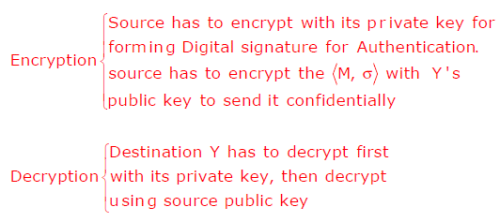Computer Science And Information Technology - CS 2013 GATE Paper (Practice Test) - Question 25

Match the problem domains in Group I with the solution technologies in Group II.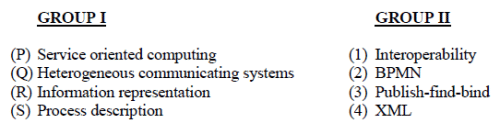Computer Science And Information Technology - CS 2013 GATE Paper (Practice Test) - Question 26

A scheduling algorithm assigns priority proportional to the waiting time of a process. Every
process starts with priority zero(the lowest priority). The scheduler re-evaluates the process
priorities every T time units and decides the next process to schedule. Which one of the
following is TRUE if the processes have no I/O operations and all arrive at time zero?

Detailed Solution for Computer Science And Information Technology - CS 2013 GATE Paper (Practice Test) - Question 26

The given scheduling definition takes two parameters, one is dynamically assigned process
priority and the other is ‘T’ time unit to re-evaluate the process priorities.
This dynamically assigned priority will be deciding processes order in ready queue of round
robin algorithm whose time quantum is same as ‘T’ time units. As all the processes are
arriving at the same time, they will be given same priority but soon after first ‘T’ time burst
remaining processes will get higher priorities

Computer Science And Information Technology - CS 2013 GATE Paper (Practice Test) - Question 27

What is the maximum number of reduce moves that can be taken by a bottom-up parser for a
grammar with no epsilon- and unit-production (i.e., of type A →and A→a) to parse a
string with n tokens?

Detailed Solution for Computer Science And Information Technology - CS 2013 GATE Paper (Practice Test) - Question 27

To have maximum number of reduce moves, all the productions will be of the typeA→αb
(where  and  could be terminals or non-terminals). Consider the following illustration
then: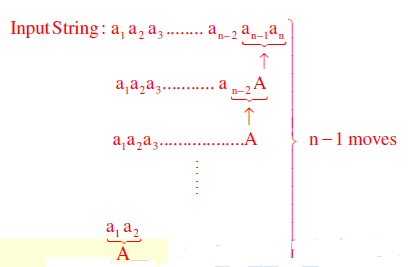Computer Science And Information Technology - CS 2013 GATE Paper (Practice Test) - Question 28

Consider the languages and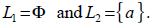. Which one of the following represents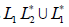?

Detailed Solution for Computer Science And Information Technology - CS 2013 GATE Paper (Practice Test) - Question 28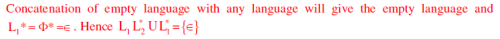Computer Science And Information Technology - CS 2013 GATE Paper (Practice Test) - Question 29

Which one of the following is the tightest upper bound that represents the time complexity of
inserting an object into a binary search tree of n nodes?

Detailed Solution for Computer Science And Information Technology - CS 2013 GATE Paper (Practice Test) - Question 29

For skewed binary search tree on n nodes, the tightest upper bound to insert a node is O(n)

Computer Science And Information Technology - CS 2013 GATE Paper (Practice Test) - Question 30

Which one of the following is the tightest upper bound that represents the number of swaps
required to sort n numbers using selection sort?

Detailed Solution for Computer Science And Information Technology - CS 2013 GATE Paper (Practice Test) - Question 30

The maximum number of swaps that takes place in selection sort on n numbers is n

Computer Science And Information Technology - CS 2013 GATE Paper (Practice Test) - Question 31

In the following truth table, V = 1 if and only if the input is valid.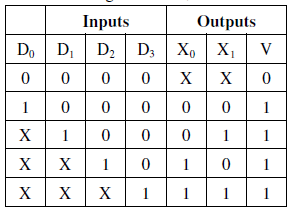What function does the truth table represent?

Detailed Solution for Computer Science And Information Technology - CS 2013 GATE Paper (Practice Test) - Question 31

4 to 2 priority encoder.

Computer Science And Information Technology - CS 2013 GATE Paper (Practice Test) - Question 32

The smallest integer than can be represented by an 8-bit number in 2’s complement form is

Detailed Solution for Computer Science And Information Technology - CS 2013 GATE Paper (Practice Test) - Question 32

−28−1 = −128 . Range is -2(n-1) to +2(n-1)-1

Computer Science And Information Technology - CS 2013 GATE Paper (Practice Test) - Question 33

Which one of the following does NOT equal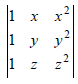Detailed Solution for Computer Science And Information Technology - CS 2013 GATE Paper (Practice Test) - Question 33

If matrix B is obtained from matrix A by replacing the lth row by itself plus k times the mth row, for l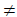m then det(B)=det(A). With this property given matrix is equal to the matrices
given in options (B),(C) and (D).

Computer Science And Information Technology - CS 2013 GATE Paper (Practice Test) - Question 34

Suppose p is number of cars per minute passing through a certain road junction between 5
PM and 6PM, and p has a Poisson distribution with mean 3. What is the probability of
observing fewer than 3 cars during any given minute in this interval?

Detailed Solution for Computer Science And Information Technology - CS 2013 GATE Paper (Practice Test) - Question 34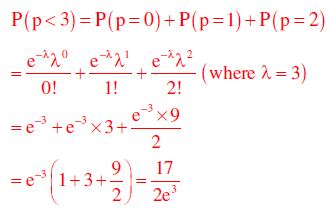Computer Science And Information Technology - CS 2013 GATE Paper (Practice Test) - Question 35

A binary operation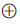on a set of integers is defined as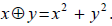. Which one of the
following statements is TRUE about?

Detailed Solution for Computer Science And Information Technology - CS 2013 GATE Paper (Practice Test) - Question 35Computer Science And Information Technology - CS 2013 GATE Paper (Practice Test) - Question 36

Q. No. 36 – 65 Carry Two Marks Each

Q.

Which one of the following is NOT logically equivalent to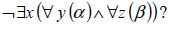Detailed Solution for Computer Science And Information Technology - CS 2013 GATE Paper (Practice Test) - Question 36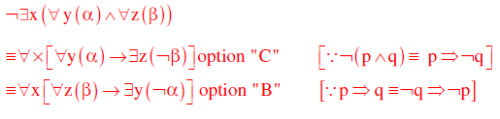Computer Science And Information Technology - CS 2013 GATE Paper (Practice Test) - Question 37

A RAM chip has a capacity of 1024 words of 8 bits each (1K x 8) . The number of 2 x 4
decoders with enable line needed to construct a 16K x 16 RAM from1K x 8 RAM is

Detailed Solution for Computer Science And Information Technology - CS 2013 GATE Paper (Practice Test) - Question 37

RAM chip size = 1k ×8[1024 words of 8 bits each]
RAM to construct =16k ×16
Number of chips required = (16K x 16)/16 x 2 [16 chips vertically with each having 2 chips horizontally]
So to select one chip out of 16 vertical chips, we need 4 x 16 decoder.
Available decoder is – 2 x 4 decoder
To be constructed is 4 x 16 decoder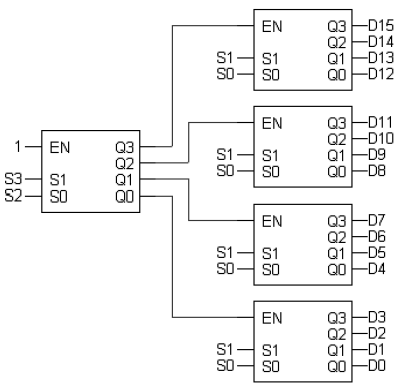So we need 5, 2 x 4 decoder in total to construct 4 x 16 decoder.

Computer Science And Information Technology - CS 2013 GATE Paper (Practice Test) - Question 38

Consider an instruction pipeline with five stages without any branch prediction: Fetch
Instruction (FI), Decode Instruction (DI), Fetch Operand (FO), Execute Instruction (EI) and
Write Operand (WO). The stage delays for FI, DI, FO, EI and WO are 5 ns, 7 ns, 10 ns, 8 ns
and 6 ns, respectively. There are intermediate storage buffers after each stage and the delay of
each buffer is 1 ns. A program consisting of I2 instructions  I1 , I2 , I3 ,......I is executed in this
pipelined processor. Instruction  I4 is the only branch instruction and its branch target is 9 I . If
the branch is taken during the execution of this program, the time (in ns) needed to complete
the program is

Detailed Solution for Computer Science And Information Technology - CS 2013 GATE Paper (Practice Test) - Question 38

Clock period=Maximum stage delay+ overhead (Buffer) =10+1=11 ns
Assume FI-1, DI-2, FO-3, EI-4, WO-5So number of clocks required to complete the program is = 15 clocks and time taken is = 15 ×11 ns=165 ns.

Computer Science And Information Technology - CS 2013 GATE Paper (Practice Test) - Question 39

Consider the following operation along with Enqueue and Dequeue operations on queues,
where k is a global parameter

MultiDequeue Q {
m k
while Q is not empty and m 0 {
Dequeue Q
m m 1
}
}

What is the worst case time complexity of a sequence of n queue operations on an initially
empty queue?

Detailed Solution for Computer Science And Information Technology - CS 2013 GATE Paper (Practice Test) - Question 39

Initially the queue is empty and we have to perform n operations.
i) One option is to perform all Enqueue operations i.e. n Enqueue operations. Complexity
will be (n)
or
ii) We can perform a mix of Enqueue and Dequeue operations. It can be Enqueue for first
n/2 times and then Dequeue for next n/2, or Enqueue and Dequeue alternately, or any
permutation of Enqueues and Dequeues totaling ‘n’ times. Complexity will be (n)
or
iii) We can perform Enqueues and MultiDequeues. A general pattern could be as follows:

Enqueue Enqueue … (ktimes) MultiDequeue Enqueue Enqueue … (ktimes) MultiDequeue
… Up to total n
---- k items enqueued -----k items deleted----k items enqueued----k items deleted -- and
so on.
The number of times this k-Enqueues, MutiDequeue cycle is performed = n/k +1
So, Complexity will be k times Enqueue + 1 MultiDequeue) x n/k+1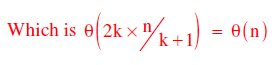or
iv) We can just perform n MultiDequeues (or n Dequeues for that matter):
Each time the while condition is false (empty queue), condition is checked just once for
each of the ‘n’ operations. So θ(n).

Computer Science And Information Technology - CS 2013 GATE Paper (Practice Test) - Question 40

The preorder traversal sequence of a binary search tree is 30, 20, 10, 15, 25, 23, 39, 35, 42.
Which one of the following is the postorder traversal sequence of the same tree?

Detailed Solution for Computer Science And Information Technology - CS 2013 GATE Paper (Practice Test) - Question 40

Pr eorder :30,20,10,15,25,23,39,35,42
Inorder :10,15,20,23,25,30,35,39,42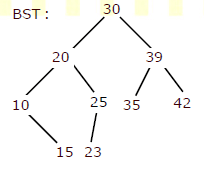Computer Science And Information Technology - CS 2013 GATE Paper (Practice Test) - Question 41

What is the return value of  f(p,p) if the value of p is initialized to 5 before the call? Note
that the first parameter is passed by reference, whereas the second parameter is passed by
value.
int f (int &x, int c) {
c= c- 1;
if (c= 0) return 1;
x = x+ 1;
return f (x,c )* x;
}

Detailed Solution for Computer Science And Information Technology - CS 2013 GATE Paper (Practice Test) - Question 41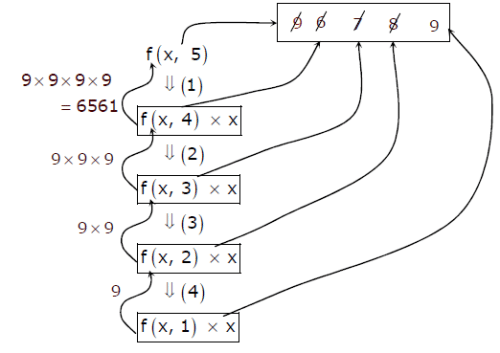Computer Science And Information Technology - CS 2013 GATE Paper (Practice Test) - Question 42

Which of the following is/are undecidable?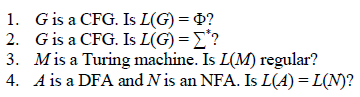Detailed Solution for Computer Science And Information Technology - CS 2013 GATE Paper (Practice Test) - Question 42

There is an algorithm to check whether the given CFG is empty, finite or infinite and also to convert NFA to DFA hence 1 and 4 are decidable

Computer Science And Information Technology - CS 2013 GATE Paper (Practice Test) - Question 43

Consider the following two sets of LR(1) items of an LR(1) grammar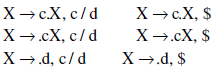Which of the following statements related to merging of the two sets in the corresponding
LALR parser is/are FALSE?

1.  Cannot be merged since look aheads are different
2. Can be merged but will result in S–R conflict
3. Can be merged but will result in R–R conflict
4. Cannot be merged since goto on c will lead to two different sets

Detailed Solution for Computer Science And Information Technology - CS 2013 GATE Paper (Practice Test) - Question 43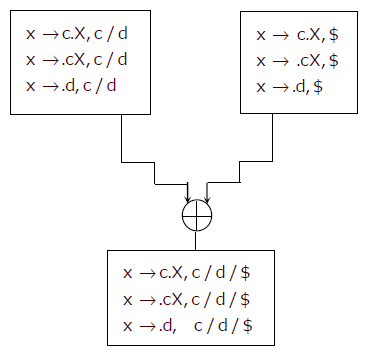1. Merging of two states depends on core part (production rule with dot operator), not on look aheads.
2. The two states are not containing Reduce item ,So after merging, the merged state can not contain any S-R conflict
3. As there is no Reduce item in any of the state, so can’t have R-R conflict.
4. Merging of stats does not depend on further goto on any terminal.
So all statements are false.

Computer Science And Information Technology - CS 2013 GATE Paper (Practice Test) - Question 44

A certain computation generates two arrays a and b such that a[i] = f (i) for 0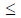i < n and
b[i] = g (a [i]) for 0i < n . Suppose this computation is decomposed into two concurrent
processes X and Y such that X computes the array a and Y computes the array b. The
processes employ two binary semaphores R and S, both initialized to zero. The array a is
shared by the two processes. The structures of the processes are shown below.

Process X:
private i;
for (i=0; i<n; i++) {
a[i] = f(i);
ExitX(R, S);
}

Process Y:
private i;
for (i=0; i<n; i++) {
EntryY(R, S);
b[i] = g(a[i]);
}

Which one of the following represents the CORRECT implementations of ExitX and EntryY?

Detailed Solution for Computer Science And Information Technology - CS 2013 GATE Paper (Practice Test) - Question 44

For computing both the array a[] and b[], first element a[i] should be computed using which b[i] can be computed. So process X and Y should run in strict alteration manner, starting with X. This requirement meets with implementation of ExitX and EntryY given in option C.

Computer Science And Information Technology - CS 2013 GATE Paper (Practice Test) - Question 45

The following figure represents access graphs of two modules M1 and M2. The filled circles
represent methods and the unfilled circles represent attributes. IF method m is moved to
module M2 keeping the attributes where they are, what can we say about the average
cohesion and coupling between modules in the system of two modules?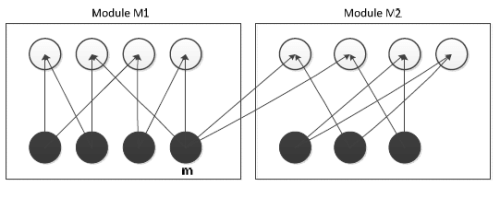Detailed Solution for Computer Science And Information Technology - CS 2013 GATE Paper (Practice Test) - Question 45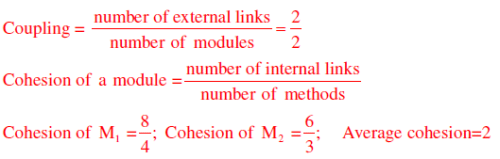After moving method m to M2, graph will become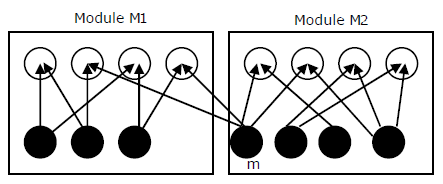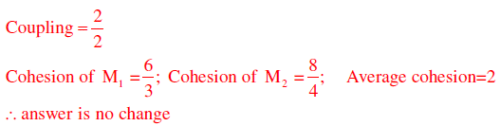Computer Science And Information Technology - CS 2013 GATE Paper (Practice Test) - Question 46

In an IPv4 datagram, the M bit is 0, the value of HLEN is 10, the value of total length is 400
and the fragment offset value is 300. The position of the datagram, the sequence numbers of
the first and the last bytes of the payload, respectively are

Detailed Solution for Computer Science And Information Technology - CS 2013 GATE Paper (Practice Test) - Question 46

M= 0 – Means there is no fragment after this, i.e. Last fragment
HLEN=10 - So header length is 4×10=40, as 4 is constant scale factor
Fragment Offset = 300, that means 300×8 Byte = 2400 bytes are before this last fragment
So the position of datagram is last fragment
Sequence number of First Byte of Payload = 2400 (as 0 to 2399 Sequence no are used)
Sequence number of Last Byte of Payload = 2400+360-1=2759

Computer Science And Information Technology - CS 2013 GATE Paper (Practice Test) - Question 47

Determine the maximum length of cable (in km) for transmitting data at a rate of 500 Mbps in
an Ethernet LAN with frames of size 10,000 bits. Assume the signal speed in the cable to be
2,00,000 km/s

Detailed Solution for Computer Science And Information Technology - CS 2013 GATE Paper (Practice Test) - Question 47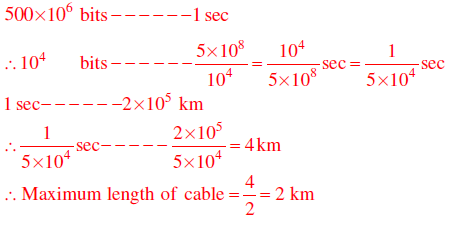Computer Science And Information Technology - CS 2013 GATE Paper (Practice Test) - Question 48

Consider the following relational schema.
Students(rollno: integer, sname: string)
Courses(courseno: integer, cname: string)
Registration(rollno: integer, courseno; integer, percent: real)
Which of the following queries are equivalent to this query in English?
“Find the distinct names of all students who score more than 90% in the course numbered
107”
(I) SELECT DISTINCT S.sname
FROM Students as S, Registration as R
WHERE R.rollno=S.rollno AND R.Courseno=107 AND R.percent>90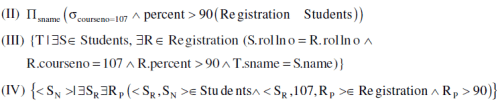Detailed Solution for Computer Science And Information Technology - CS 2013 GATE Paper (Practice Test) - Question 48

Four queries given in SQL, RA, TRC and DRC in four statements respectively retrieve the required information.

Computer Science And Information Technology - CS 2013 GATE Paper (Practice Test) - Question 49

A shared variable x, initialized to zero, is operated on by four concurrent processes W, X, Y, Z as
follows. Each of the processes W and X reads x from memory, increments by one, stores it to
memory, and then terminates. Each of the processes Y and Z reads x from memory, decrements by
two, stores it to memory, and then terminates. Each process before reading x invokes the P
operation (i.e., wait) on a counting semaphore S and invokes the V operation (i.e., signal) on the
semaphore S after storing x to memory. Semaphore S is initialized to two. What is the maximum
possible value of x after all processes complete execution?

Detailed Solution for Computer Science And Information Technology - CS 2013 GATE Paper (Practice Test) - Question 49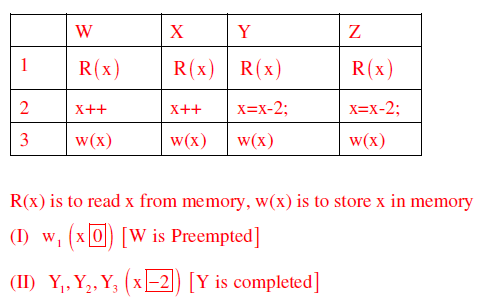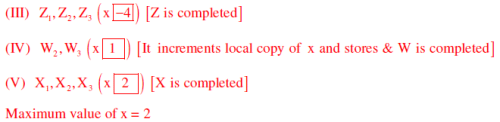Computer Science And Information Technology - CS 2013 GATE Paper (Practice Test) - Question 50

Consider the DFA given below.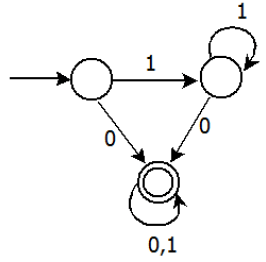Which of the following are FALSE?

1. Complement of L(A) is context–free

2.  L(A) = L((11* 0 + 0)(0 +1)* 0 *1*)
3. For the language accepted by A, A is the minimal DFA
4. A accepts all strings over {0, 1} of length at least 2

Detailed Solution for Computer Science And Information Technology - CS 2013 GATE Paper (Practice Test) - Question 50

(1) L(A) is regular, its complement is also regular and if it is regular it is also context free.
(2) L(A) =(11*0 + 0)(0 +1)* 0*1* = 1*0 (0 +1)*
Language has all strings where each string contains ‘0’.
(3) A is not minimal, it can be constructed with 2 states
(4) Language has all strings, where each string contains ‘0’. (atleast length one)

Computer Science And Information Technology - CS 2013 GATE Paper (Practice Test) - Question 51

Consider the following languages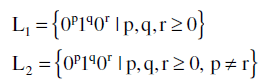Which one of the following statements is FALSE?

Detailed Solution for Computer Science And Information Technology - CS 2013 GATE Paper (Practice Test) - Question 51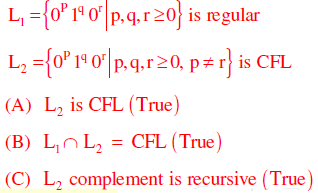(D) L1 complement is CFL but not regular False as L1 is regular L1 is regular

Computer Science And Information Technology - CS 2013 GATE Paper (Practice Test) - Question 52

Consider the following function

int unknown (int n) {
int i, j, k 0;
for (i =n/2; i<= n; i++)
for (j= 2; j<= n; j= j* 2)
k= k+ n / 2;
return k ;
}
The return value of the function is

Detailed Solution for Computer Science And Information Technology - CS 2013 GATE Paper (Practice Test) - Question 52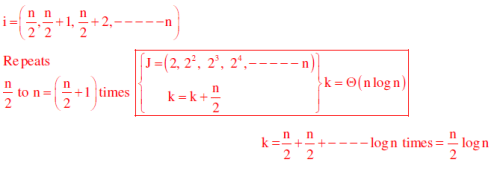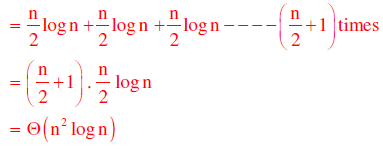Computer Science And Information Technology - CS 2013 GATE Paper (Practice Test) - Question 53

The number of elements that can be sorted in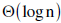time using heap sort is

Detailed Solution for Computer Science And Information Technology - CS 2013 GATE Paper (Practice Test) - Question 53

After constructing a max-heap in the heap sort , the time to extract maximum element and
then heapifying the heap takes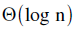time by which we could say thattime is
required to correctly place an element in sorted array. Iftime is taken to sort using
heap sort, then number of elements that can be sorted is constant which is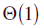Computer Science And Information Technology - CS 2013 GATE Paper (Practice Test) - Question 54

Consider a hard disk with 16 recording surfaces (0 −15) having 16384 cylinders (0 −16383)
and each cylinder contains 64 sectors (0 − 63) . Data storage capacity in each sector is 512
bytes. Data are organized cylinder–wise and the addressing format is <cylinder no., sector
no.>. A file of size 42797 KB is stored in the disk and the starting disk location of the file is
<1200, 9, 40>. What is the cylinder number of the last sector of the file, if it is stored in a
contiguous manner?

Detailed Solution for Computer Science And Information Technology - CS 2013 GATE Paper (Practice Test) - Question 54

42797 KB=(42797x 1024 )/512= 85594 sectors

Starting is 1200,9,40 contains total 24 + (6×64) = 408 sectors
Next, 1201, --------, 1283 cylinders contains total 1024×83 = 84992 sec tors
(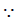each cylinder contains 16 64 1024 sec tors)Total 408 + 84992= 85400 sec torsThe required cylinder number is 1284 which will contain the last sector of the file

Computer Science And Information Technology - CS 2013 GATE Paper (Practice Test) - Question 55

Consider the following sequence of micro–operations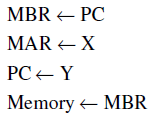Which one of the following is a possible operation performed by this sequence?

Detailed Solution for Computer Science And Information Technology - CS 2013 GATE Paper (Practice Test) - Question 55

PC content is stored in memory via MBR and PC gets new address from Y. It represents a
function call (routine), which is matching with interrupt service initiation

Computer Science And Information Technology - CS 2013 GATE Paper (Practice Test) - Question 56

The line graph L(G) of a simple graph G is defined as follows:
• There is exactly one vertex v(e) in L(G) for each edge e in G.
• For any two edges e and e’ in G, L(G) has an edge between v(e) and v(e’), if and only if e
and e’ are incident with the same vertex in G.
Which of the following statements is/are TRUE?

(P) The line graph of a cycle is a cycle.
(Q) The line graph of a clique is a clique.
(R) The line graph of a planar graph is planar.
(S) The line graph of a tree is a tree.

Detailed Solution for Computer Science And Information Technology - CS 2013 GATE Paper (Practice Test) - Question 56

P) The line graph of a cycle is a cycle

R) Line graph of planar graph need not be planar always. Consider the following example. Consider the following planar graph (star graph)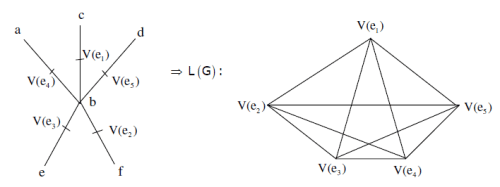S) Hence line graph of planar graph need not be planar(Here we got K5 which is not planar).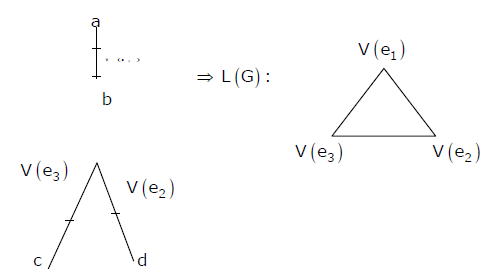The line graph of a tree need not be tree.

Computer Science And Information Technology - CS 2013 GATE Paper (Practice Test) - Question 57

What is the logical translation of the following statement?
“None of my friends are perfect.”

Computer Science And Information Technology - CS 2013 GATE Paper (Practice Test) - Question 58

The procedure given below is required to find and replace certain characters inside an input
character string supplied in array A. The characters to be replaced are supplied in array oldc,
while their respective replacement characters are supplied in array newc. Array A has a fixed
length of five characters, while arrays oldc and newc contain three characters each. However, the
procedure is flawed

void find _ and _ replace (char *A, char *oldc, char * newc ) {
for (int i= 0; i <5; i++)
for (int j =0; j <3; j++)
if (A [i] ==oldc[ j])  A [i]= newc [j] ;
}
The procedure is tested with the following four test cases
(1) oldc = "abc", newc = "dab"            (2) oldc = "cde", newc = "bcd"
(3) oldc = "bca", newc = "cda"            (4) oldc = "abc", newc = "bac"

Q.

The tester now tests the program on all input strings of length five consisting of characters
‘a’, ‘b’, ‘c’, ‘d’ and ‘e’ with duplicates allowed. If the tester carries out this testing with the
four test cases given above, how many test cases will be able to capture the flaw?

Detailed Solution for Computer Science And Information Technology - CS 2013 GATE Paper (Practice Test) - Question 58

Flaw in this given procedure is that one character of Array ‘A’ can be replaced by more than one character of newc array, which should not be so.Test case (3) and (4) identifies this flaw as they are containing ‘oldc’ and ‘newc’ array characters arranged in specific manner.
Following string can reflect flaw, if tested by test case (3). initially i = j = 0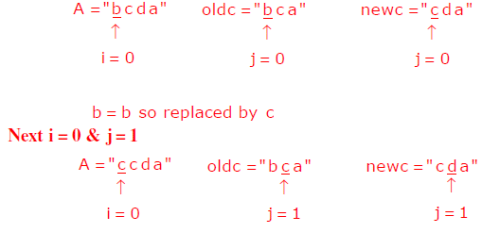c = c so replaced by d

Likewise single character ‘b’ in A is replaced by ‘c’ and then by ‘d’.
Same way test case (4) can also catch the flaw

Computer Science And Information Technology - CS 2013 GATE Paper (Practice Test) - Question 59

The procedure given below is required to find and replace certain characters inside an input
character string supplied in array A. The characters to be replaced are supplied in array oldc,
while their respective replacement characters are supplied in array newc. Array A has a fixed
length of five characters, while arrays oldc and newc contain three characters each. However, the
procedure is flawed

void find _ and _ replace (char *A, char *oldc, char * newc ) {
for (int i= 0; i <5; i++)
for (int j =0; j <3; j++)
if (A [i] ==oldc[ j])  A [i]= newc [j] ;
}
The procedure is tested with the following four test cases
(1) oldc = "abc", newc = "dab"            (2) oldc = "cde", newc = "bcd"
(3) oldc = "bca", newc = "cda"            (4) oldc = "abc", newc = "bac"

Q.

If array A is made to hold the string “abcde”, which of the above four test cases will be
successful in exposing the flaw in this procedure?

Detailed Solution for Computer Science And Information Technology - CS 2013 GATE Paper (Practice Test) - Question 59

Now for string “abcde” in array A, both test case (3) and (4) will be successful in finding the
flaw, as explained in above question.

Computer Science And Information Technology - CS 2013 GATE Paper (Practice Test) - Question 60

The following code segment is executed on a processor which allows only register operands in
its instructions. Each instruction can have almost two source operands and one destination operand.
Assume that all variables are dead after this code segment

c= a+ b;
d = c * a;
e= c+ a;
x =c *c;
if ( x >a) {
y= a * a;
}
else {
d =d * d;
e =e * e;
}

Q.

Suppose the instruction set architecture of the processor has only two registers. The only allowed
compiler optimization is code motion, which moves statements from one place to another while
preserving correctness. What is the minimum number of spills to memory in the compiled code?

Computer Science And Information Technology - CS 2013 GATE Paper (Practice Test) - Question 61

The following code segment is executed on a processor which allows only register operands in
its instructions. Each instruction can have almost two source operands and one destination operand.
Assume that all variables are dead after this code segment

c= a+ b;
d = c * a;
e= c+ a;
x =c *c;
if ( x >a) {
y= a * a;
}
else {
d =d * d;
e =e * e;
}

Q.

What is the minimum number of registers needed in the instruction set architecture of the
processor to compile this code segment without any spill to memory? Do not apply any
optimization other than optimizing register allocation

Detailed Solution for Computer Science And Information Technology - CS 2013 GATE Paper (Practice Test) - Question 61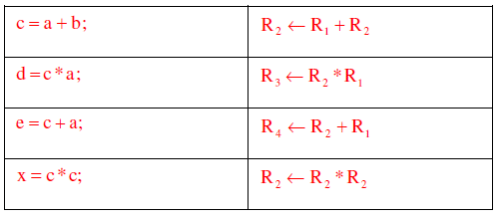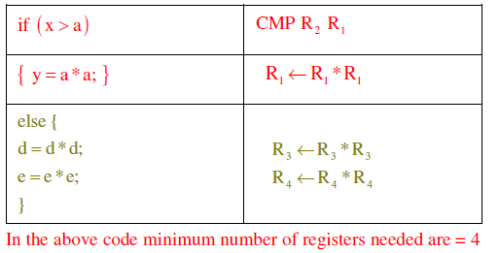Computer Science And Information Technology - CS 2013 GATE Paper (Practice Test) - Question 62

Relation R has eight attributes ABCDEFGH. Fields of R contain only atomic values.
F ={CH→G, A→BC, B→CFH, E→A, F→EG} is a set of functional dependencies (FDs) so that F+ is exactly the set of FDs that hold for R

Q.

How many candidate keys does the relation R have?

Detailed Solution for Computer Science And Information Technology - CS 2013 GATE Paper (Practice Test) - Question 62

Candidate keys are AD, BD, ED and FD

Computer Science And Information Technology - CS 2013 GATE Paper (Practice Test) - Question 63

Relation R has eight attributes ABCDEFGH. Fields of R contain only atomic values.
F ={CH→G, A→BC, B→CFH, E→A, F→EG} is a set of functional dependencies (FDs) so that F+ is exactly the set of FDs that hold for R

Q.

The relation R is

Detailed Solution for Computer Science And Information Technology - CS 2013 GATE Paper (Practice Test) - Question 63

A→BC,B→CFH and F→EG are partial dependencies. Hence it is in 1NF but not in 2NF

Computer Science And Information Technology - CS 2013 GATE Paper (Practice Test) - Question 64

A computer uses 46–bit virtual address, 32–bit physical address, and a three–level paged page
table organization. The page table base register stores the base address of the first–level table
( T1) ,which occupies exactly one page. Each entry of T1 stores the base address of a page of the
second–level table ( T2) . Each entry of T2 stores the base address of a page of the third–level table
( T3)  Each entry of T3 stores a page table entry (PTE). The PTE is 32 bits in size. The processor
used in the computer has a 1 MB 16 way set associative virtually indexed physically tagged cache.
The cache block size is 64 bytes.

Q.

What is the size of a page in KB in this computer?

Detailed Solution for Computer Science And Information Technology - CS 2013 GATE Paper (Practice Test) - Question 64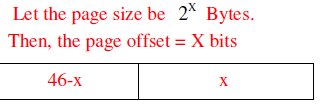Now, we are using 3-level paging. First level page table is contained in one page. Each page table entry is 32-bit.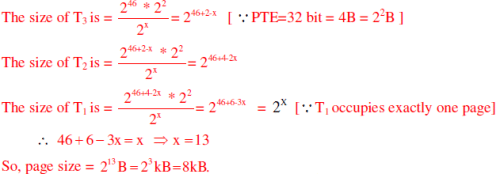Computer Science And Information Technology - CS 2013 GATE Paper (Practice Test) - Question 65

A computer uses 46–bit virtual address, 32–bit physical address, and a three–level paged page
table organization. The page table base register stores the base address of the first–level table
( T1) ,which occupies exactly one page. Each entry of T1 stores the base address of a page of the
second–level table ( T2) . Each entry of T2 stores the base address of a page of the third–level table
( T3)  Each entry of T3 stores a page table entry (PTE). The PTE is 32 bits in size. The processor
used in the computer has a 1 MB 16 way set associative virtually indexed physically tagged cache.
The cache block size is 64 bytes.

Q.

What is the minimum number of page colours needed to guarantee that no two synonyms map to
different sets in the processor cache of this computer?

Detailed Solution for Computer Science And Information Technology - CS 2013 GATE Paper (Practice Test) - Question 65

As the page size is  213 Bytes and page coloring is asked so we divide cache size by page size and group 16 pages in one set.
Number of pages in cache=1MB/8KB=128 pages

Number of set in cache=128/16=8 sets

Take any page of LAS, it will be mapped with cache on any one of these 8 sets (set
association mapping).For any two synonym to map with same set they should be colored with same color of that respective set. So minimum we need 8 colors for this mapping.

## GATE Past Year Papers for Practice (All Branches)

407 docs|127 tests
Information about Computer Science And Information Technology - CS 2013 GATE Paper (Practice Test) Page
In this test you can find the Exam questions for Computer Science And Information Technology - CS 2013 GATE Paper (Practice Test) solved & explained in the simplest way possible. Besides giving Questions and answers for Computer Science And Information Technology - CS 2013 GATE Paper (Practice Test), EduRev gives you an ample number of Online tests for practice

## GATE Past Year Papers for Practice (All Branches)

407 docs|127 tests(Scan QR code)